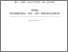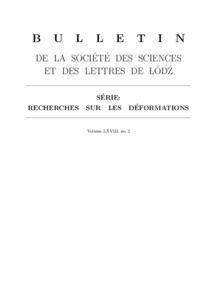# Integral theorems for monogenic functions in an infinite-dimensional space with a commutative multiplication

Плакса, С. А. and Шпаківський, В. С. (2018) Integral theorems for monogenic functions in an infinite-dimensional space with a commutative multiplication. Recherches sur les d´eformations, 2 (LXVIII). pp. 25-36.Preview
Text

## Abstract

We consider monogenic functions taking values in a topological vector space being an expansion of a certain infinite-dimensional commutative Banach algebra associated with the three-dimensional Laplace equation. We establish also integral theorems for monogenic functions taking values in the mentioned algebra and the mentioned topological vector space.

Item Type: Article Laplace equation, spatial potentials, harmonic algebra, topological vector space, differentiable in the sense of Gˆateaux function, monogenic function, Cauchy- Riemann conditions Q Science > QA Mathematics > Mathematical Analysis Faculty of Physics and Mathematics > Department of Mathematical Analysis Ірина Ігорівна Таргонська 26 Feb 2019 09:40 26 Feb 2019 09:49 http://eprints.zu.edu.ua/id/eprint/28414View Item